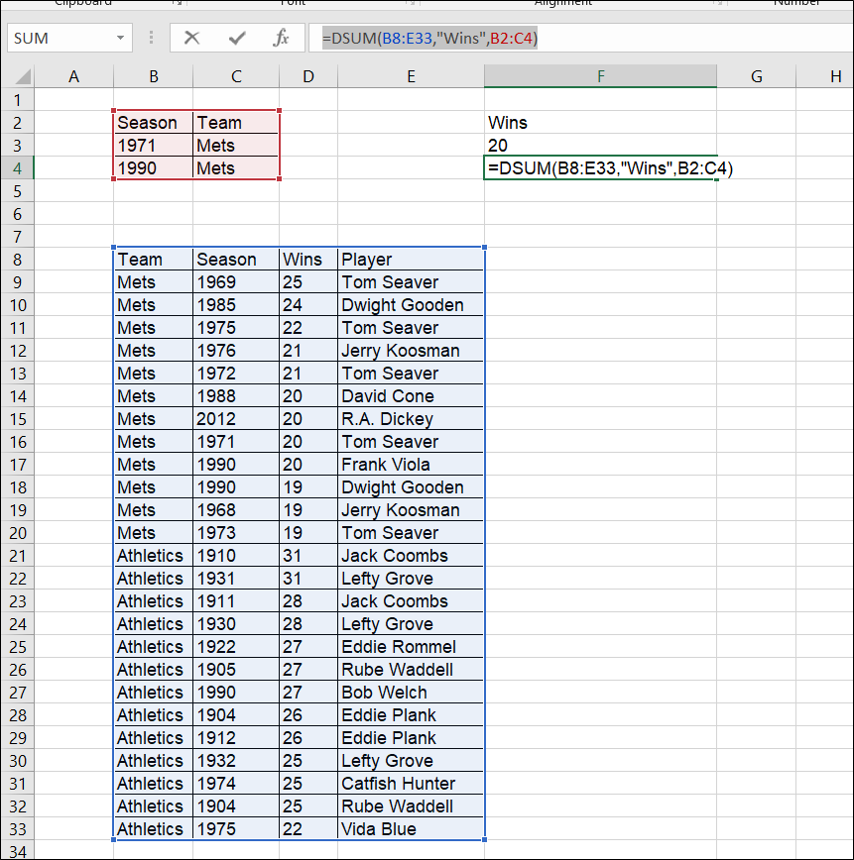Search

# Excel DSUM formula

Excel's DSUM formula is a useful alternative to VLOOKUP. It will read the column headings of an array of data, and return the sum based on criteria entered in separate set of cells.

In this example, the DSUM formula:

=DSUM(B8:E33,"Wins",B2:C4)

. . . makes references to the data in B8:E33, and is set to add up the numbers in the 'Wins' column. The array given at the end of the formula checks the criteria to use in filtering down the numbers to add up. So the result will be 59, the number of wins for the listed Mets pitchers in 1971 and 1990.